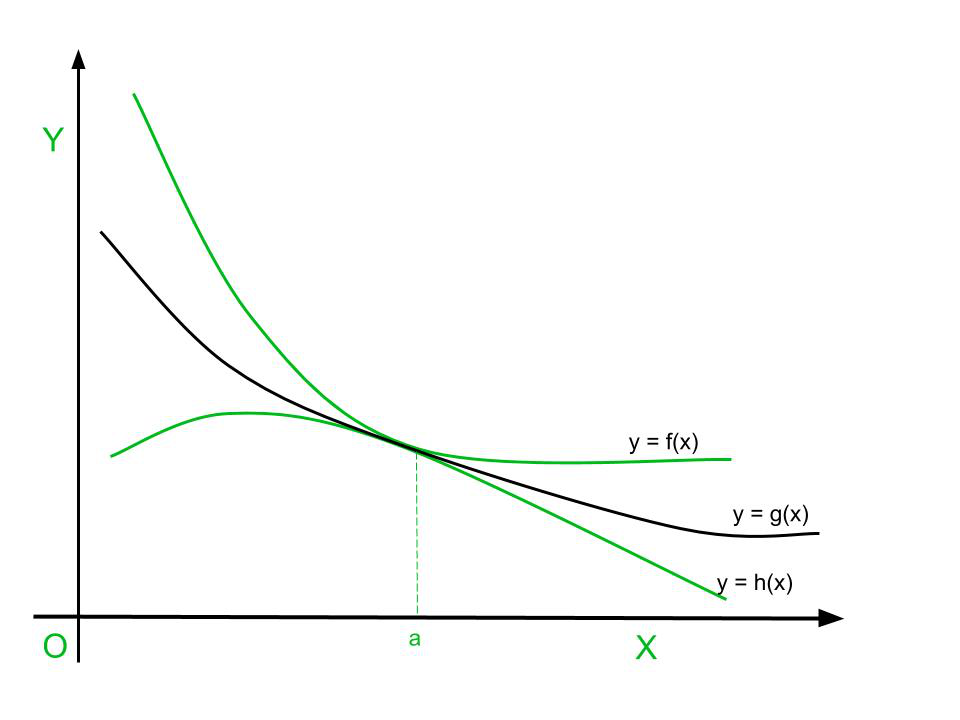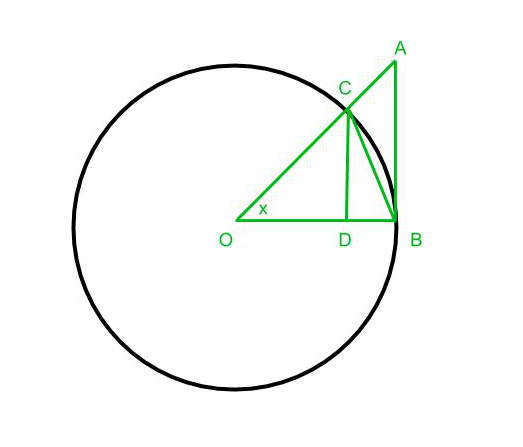# Squeeze Theorem – Limits

• Difficulty Level : Expert
• Last Updated : 12 May, 2021

Squeeze theorem is also known as the sandwich theorem, the sandwich rule, the police theorem, the pinching theorem sometimes the squeeze lemma, the theorem of carabinieri is used in mathematical analysis to find the limit of a function when there are two other functions whose limits are known are present. The squeeze theorem is applied in calculus and mathematical analysis. It is typically applied to confirm the limit of a function via comparison with two other functions whose limits are known or easily computed. It was first used geometrically by the mathematicians Archimedes and Eudoxus in an effort to compute π and was formulated in modern terms by Carl Friedrich Gauss.

## Theorem

The squeeze theorem is formally stated as follows:

Let functions f(x), g(x), h(x) be real functions such that h(x) ≤ g(x) ≤ f(x). Then for all x in the domain of the function,Here the functions h and f are said to be lower and upper bounds of g respectively. The above function is represented in a graph below.## Important InequalityProof:

Consider the following image:In the diagram above O is the centre of the circle AB is a tangent of the circle from point B and CD is perpendicular to OB and angle COD is x radiansby taking the reciprocals we get## Important LimitsProof:

From the above inequality, we proved thatFrom this equation, we understand that (sin x/x) always lies between cos x and 1. So (sin x/x) is sandwiched between 1 and cos x. We know thatProof:

To prove this limit we use the trigonometric identityMy Personal Notes arrow_drop_up